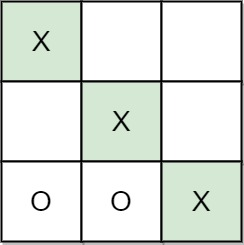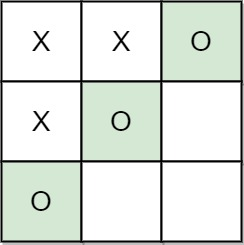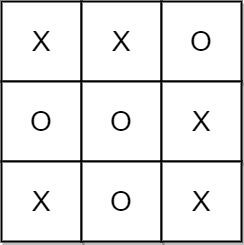# Last 6 Months Questions

Given an array of numbers(positive and negative) return the top k most combination sum(sorted)

Sample case
[5,3,-2]

possible combinations sums: 5, 3, -2, 8, 6, 1, ..You are given a non-negative integer array `nums`. In one operation, you must:

• Choose a positive integer `x` such that `x` is less than or equal to the smallest non-zero element in `nums`.
• Subtract `x` from every positive element in `nums`.

Return the minimum number of operations to make every element in `nums` equal to `0`.

Example 1:

```Input: nums = [1,5,0,3,5]
Output: 3
Explanation:
In the first operation, choose x = 1. Now, nums = [0,4,0,2,4].
In the second operation, choose x = 2. Now, nums = [0,2,0,0,2].
In the third operation, choose x = 2. Now, nums = [0,0,0,0,0].
```

Example 2:

```Input: nums = 
Output: 0
Explanation: Each element in nums is already 0 so no operations are needed.
```

Constraints:

• `1 <= nums.length <= 100`
• `0 <= nums[i] <= 100`

Give you a list servers. Their processing power is given as a array of integer, and boot power as a array of integer.
Write a function to return the max length of sub array which’s power consumption is less than or equal to max power limit.
Formula to calculate the power consumption for a subArray is:
Max(bootPower[i…j]) + Sum(processPower[i….j]) * length of subArray.

Note: Single server is also a subArray, return 0 if no such subArray can be found.

``````public int MaxLengthValidSubArray(int[] processingPower, int[] bootingPower, int maxPower)
{}``````

As the ruler of a kingdom, you have an army of wizards at your command.

You are given a 0-indexed integer array `strength`, where `strength[i]` denotes the strength of the `ith` wizard. For a contiguous group of wizards (i.e. the wizards’ strengths form a subarray of `strength`), the total strength is defined as the product of the following two values:

• The strength of the weakest wizard in the group.
• The total of all the individual strengths of the wizards in the group.

Return the sum of the total strengths of all contiguous groups of wizards. Since the answer may be very large, return it modulo `109 + 7`.

subarray is a contiguous non-empty sequence of elements within an array.

Example 1:

```Input: strength = [1,3,1,2]
Output: 44
Explanation: The following are all the contiguous groups of wizards:
-  from [1,3,1,2] has a total strength of min() * sum() = 1 * 1 = 1
-  from [1,3,1,2] has a total strength of min() * sum() = 3 * 3 = 9
-  from [1,3,1,2] has a total strength of min() * sum() = 1 * 1 = 1
-  from [1,3,1,2] has a total strength of min() * sum() = 2 * 2 = 4
- [1,3] from [1,3,1,2] has a total strength of min([1,3]) * sum([1,3]) = 1 * 4 = 4
- [3,1] from [1,3,1,2] has a total strength of min([3,1]) * sum([3,1]) = 1 * 4 = 4
- [1,2] from [1,3,1,2] has a total strength of min([1,2]) * sum([1,2]) = 1 * 3 = 3
- [1,3,1] from [1,3,1,2] has a total strength of min([1,3,1]) * sum([1,3,1]) = 1 * 5 = 5
- [3,1,2] from [1,3,1,2] has a total strength of min([3,1,2]) * sum([3,1,2]) = 1 * 6 = 6
- [1,3,1,2] from [1,3,1,2] has a total strength of min([1,3,1,2]) * sum([1,3,1,2]) = 1 * 7 = 7
The sum of all the total strengths is 1 + 9 + 1 + 4 + 4 + 4 + 3 + 5 + 6 + 7 = 44.
```

Example 2:

```Input: strength = [5,4,6]
Output: 213
Explanation: The following are all the contiguous groups of wizards:
-  from [5,4,6] has a total strength of min() * sum() = 5 * 5 = 25
-  from [5,4,6] has a total strength of min() * sum() = 4 * 4 = 16
-  from [5,4,6] has a total strength of min() * sum() = 6 * 6 = 36
- [5,4] from [5,4,6] has a total strength of min([5,4]) * sum([5,4]) = 4 * 9 = 36
- [4,6] from [5,4,6] has a total strength of min([4,6]) * sum([4,6]) = 4 * 10 = 40
- [5,4,6] from [5,4,6] has a total strength of min([5,4,6]) * sum([5,4,6]) = 4 * 15 = 60
The sum of all the total strengths is 25 + 16 + 36 + 36 + 40 + 60 = 213.
```

Constraints:

• `1 <= strength.length <= 105`
• `1 <= strength[i] <= 109`To assemble an Amazon robot, we are given N components labeled from 0 ~ N-1.
There are order requirements to put some components given by an array of pair [pre, post]
which means pre must be installed before post. Write a solution to determine if all the components
can be assmbled successfully.
ex [[0,1],[1,2],[2,0]] returns falseThere are `n` children standing in a line. Each child is assigned a rating value given in the integer array `ratings`.

You are giving candies to these children subjected to the following requirements:

• Each child must have at least one candy.
• Children with a higher rating get more candies than their neighbors.

Return the minimum number of candies you need to have to distribute the candies to the children.

Example 1:

```Input: ratings = [1,0,2]
Output: 5
Explanation: You can allocate to the first, second and third child with 2, 1, 2 candies respectively.
```

Example 2:

```Input: ratings = [1,2,2]
Output: 4
Explanation: You can allocate to the first, second and third child with 1, 2, 1 candies respectively.
The third child gets 1 candy because it satisfies the above two conditions.
```

Constraints:

• `n == ratings.length`
• `1 <= n <= 2 * 104`
• `0 <= ratings[i] <= 2 * 104`

Design a data structure that follows the constraints of a Least Recently Used (LRU) cache.

Implement the `LRUCache` class:

• `LRUCache(int capacity)` Initialize the LRU cache with positive size `capacity`.
• `int get(int key)` Return the value of the `key` if the key exists, otherwise return `-1`.
• `void put(int key, int value)` Update the value of the `key` if the `key` exists. Otherwise, add the `key-value` pair to the cache. If the number of keys exceeds the `capacity` from this operation, evict the least recently used key.

The functions `get` and `put` must each run in `O(1)` average time complexity.

Example 1:

```Input
["LRUCache", "put", "put", "get", "put", "get", "put", "get", "get", "get"]
[, [1, 1], [2, 2], , [3, 3], , [4, 4], , , ]
Output
[null, null, null, 1, null, -1, null, -1, 3, 4]

Explanation
LRUCache lRUCache = new LRUCache(2);
lRUCache.put(1, 1); // cache is {1=1}
lRUCache.put(2, 2); // cache is {1=1, 2=2}
lRUCache.get(1);    // return 1
lRUCache.put(3, 3); // LRU key was 2, evicts key 2, cache is {1=1, 3=3}
lRUCache.put(4, 4); // LRU key was 1, evicts key 1, cache is {4=4, 3=3}
lRUCache.get(3);    // return 3
lRUCache.get(4);    // return 4
```

Constraints:

• `1 <= capacity <= 3000`
• `0 <= key <= 104`
• `0 <= value <= 105`
• At most `2 * 105` calls will be made to `get` and `put`.

Design a parking system for a parking lot. The parking lot has three kinds of parking spaces: big, medium, and small, with a fixed number of slots for each size.

Implement the `ParkingSystem` class:

• `ParkingSystem(int big, int medium, int small)` Initializes object of the `ParkingSystem` class. The number of slots for each parking space are given as part of the constructor.
• `bool addCar(int carType)` Checks whether there is a parking space of `carType` for the car that wants to get into the parking lot. `carType` can be of three kinds: big, medium, or small, which are represented by `1``2`, and `3` respectively. A car can only park in a parking space of its `carType`. If there is no space available, return `false`, else park the car in that size space and return `true`.

Example 1:

```Input
[[1, 1, 0], , , , ]
Output
[null, true, true, false, false]

Explanation
ParkingSystem parkingSystem = new ParkingSystem(1, 1, 0);
parkingSystem.addCar(1); // return true because there is 1 available slot for a big car
parkingSystem.addCar(2); // return true because there is 1 available slot for a medium car
parkingSystem.addCar(3); // return false because there is no available slot for a small car
parkingSystem.addCar(1); // return false because there is no available slot for a big car. It is already occupied.
```

Constraints:

• `0 <= big, medium, small <= 1000`
• `carType` is `1``2`, or `3`
• At most `1000` calls will be made to `addCar`

Tic-tac-toe is played by two players `A` and `B` on a `3 x 3` grid. The rules of Tic-Tac-Toe are:

• Players take turns placing characters into empty squares `' '`.
• The first player `A` always places `'X'` characters, while the second player `B` always places `'O'` characters.
• `'X'` and `'O'` characters are always placed into empty squares, never on filled ones.
• The game ends when there are three of the same (non-empty) character filling any row, column, or diagonal.
• The game also ends if all squares are non-empty.
• No more moves can be played if the game is over.

Given a 2D integer array `moves` where `moves[i] = [rowi, coli]` indicates that the `ith` move will be played on `grid[rowi][coli]`. return the winner of the game if it exists (`A` or `B`). In case the game ends in a draw return `"Draw"`. If there are still movements to play return `"Pending"`.

You can assume that `moves` is valid (i.e., it follows the rules of Tic-Tac-Toe), the grid is initially empty, and `A` will play first.

Example 1:```Input: moves = [[0,0],[2,0],[1,1],[2,1],[2,2]]
Output: "A"
Explanation: A wins, they always play first.
```

Example 2:```Input: moves = [[0,0],[1,1],[0,1],[0,2],[1,0],[2,0]]
Output: "B"
Explanation: B wins.
```

Example 3:```Input: moves = [[0,0],[1,1],[2,0],[1,0],[1,2],[2,1],[0,1],[0,2],[2,2]]
Output: "Draw"
Explanation: The game ends in a draw since there are no moves to make.
```

Constraints:

• `1 <= moves.length <= 9`
• `moves[i].length == 2`
• `0 <= rowi, coli <= 2`
• There are no repeated elements on `moves`.
• `moves` follow the rules of tic tac toe.QUESTION:
A Z sequence is defined as:

Zi=P×X(Zi−1)+Q for i>0
Zi = 2 for i=0
X(K) is defined as the number of set bits in the binary form of a number K.
Print the number of set bits in the binary form of ZN

Example

N = 2
P = 1
Q = 3
Approach

So , Z = 2 , Z = PX+Q. Now X = 1 as 2 can be written as 10 in binary form So, Z = 11+3 = 4 . Similarly , Z = PX+Q .Now 4 can be written as 100 . So , Z = 11+3 = 4. Now answer is the number of set bits in Z = 4, so 1.

EXAMPLE:
Sample input
1
3 5 1
Sample output
1
Explanation
Based on expression, sequence will be like 2, 8, 8,…

As N=1 so Z1 is 8 Number of set bits in 8(i.e 1000) is 1

QUESTION:
You are given an array A of N integers. You need to find two integers x and y such that the sum of the absolute difference between each element of the array to one of the two chosen integers is minimal.

Determine the minimum value of the expression ∑i=1nmin(abs(a[i]−x),abs(a[i]−y)) if the chosen numbers are x and y.
Example1:
N = 4
A = [2, 3, 6, 7]
Approach

You can choose the two integers, 3 and 7.
The required sum = |2 – 3| + |3 – 3| + |6 – 7| + |7 – 7| = 1 + 0 + 0 + 1 = 2.

Example2:
Given
N = 3
A = [1, 3, 5]
Approach

You can choose the two integers, 1 and 4.
The required sum = |1 – 1| + |3 – 4| + |5 – 4| = 0 + 1 + 1 = 2.
The second test case
Example3:

Given
N = 4
A = [3, 2, 5, 11]
Approach

You can choose the two integers, 3 and 11.
The required sum = |2 – 3| + |3 – 3| + |5 – 3| + |11 – 11 |= 1 + 0 + 2 + 0 = 3.

Suppose you are given a grid of 1’s and 0’s. All adjacent 1’s are connected components.
For example, in the following case you have 2 connected components because you have two “islands” of 1’s.

``````1 1 0 0 1 1
1 0 0 0 1 1
1 0 0 0 0 0
``````

Now you have a function called `insertValue(coordinates)` which takes in a row and column and inserts a 1. The function must return the updated number of connected components. So for example:
init:

``````1 1 0 0 1 1
1 0 0 0 1 1
1 0 0 0 0 0
``````

insertValue(row=1, col=1) gives 2 connected components still because
grid is:

``````1 1 0 0 1 1
1 1 0 0 1 1
1 0 0 0 0 0
``````

insertValue(row=1, col=2) gives 2 connected components still because
grid is:

``````1 1 0 0 1 1
1 1 1 0 1 1
1 0 0 0 0 0
``````

insertValue(row=1, col=3) gives 3 connected components still because
grid is:

``````1 1 0 0 1 1
1 1 1 1 1 1
1 0 0 0 0 0``````

Given a list of cities in a 2D universe, find the number of worlds in the universe.

Any pair of cities in the same world have distance <= 10000 between them
All cities in different worlds have distance > 10000

eg:
cities -> ((0,0), (2,3), (4,2), (20000,1), (20000,3), (20002,5))
Ans: Number of worlds = 2

Harry and Potter took a word string. Harry chose a number M (less than the length of the
string) and Potter chose N (less than the length of the string). Harry will cut M alphabets
from the end of the string and then add it to the beginning and will give it to Potter. Then,
Potter will also cut N alphabets from the end of the string, add it to the beginning and then
give to Harry. This process will continue till they get the original word string back.
For a given string and given values of M and N, find the number of turns in which they will
get the original word string back.

Input Specification:
input1: Original word string
Value of M​• Given a database of amazon prime video, implements following 3 functionalities :
1. Get top 10 most watched videos.
2. Watch a video
3. Get a rank of a particular video